Learn all Concepts of Polynomials Class 9 (with VIDEOS). Check - Polynomials Class 9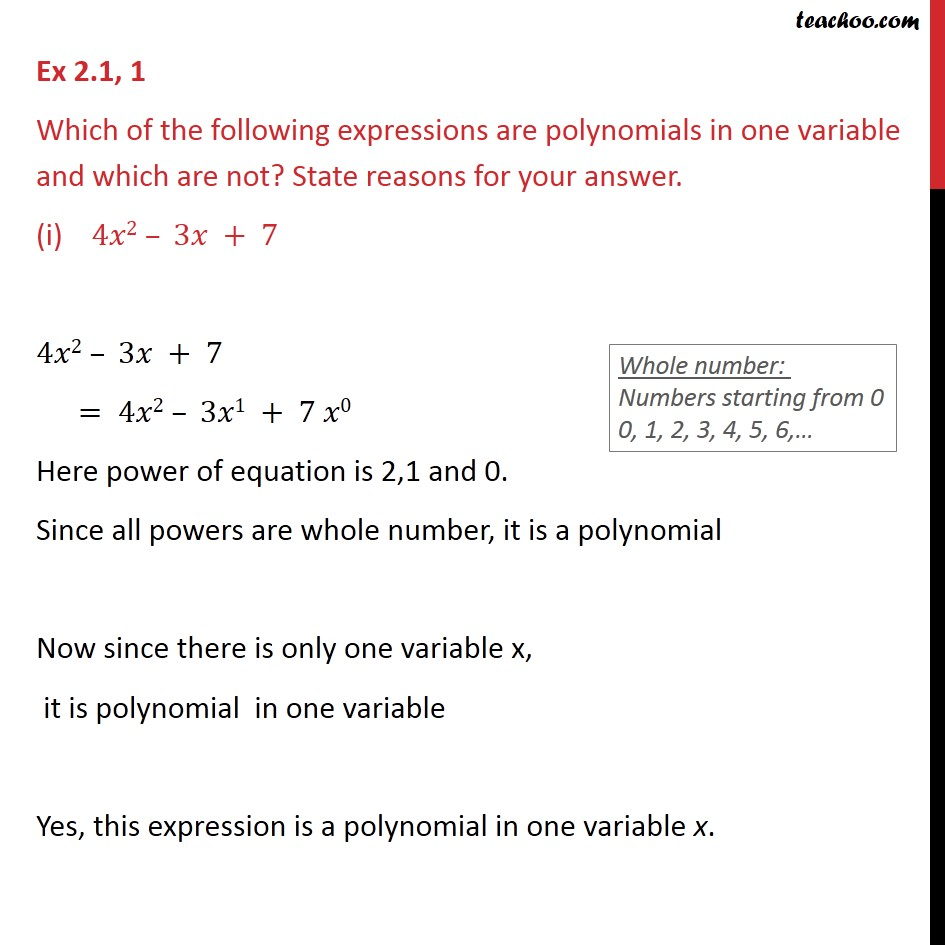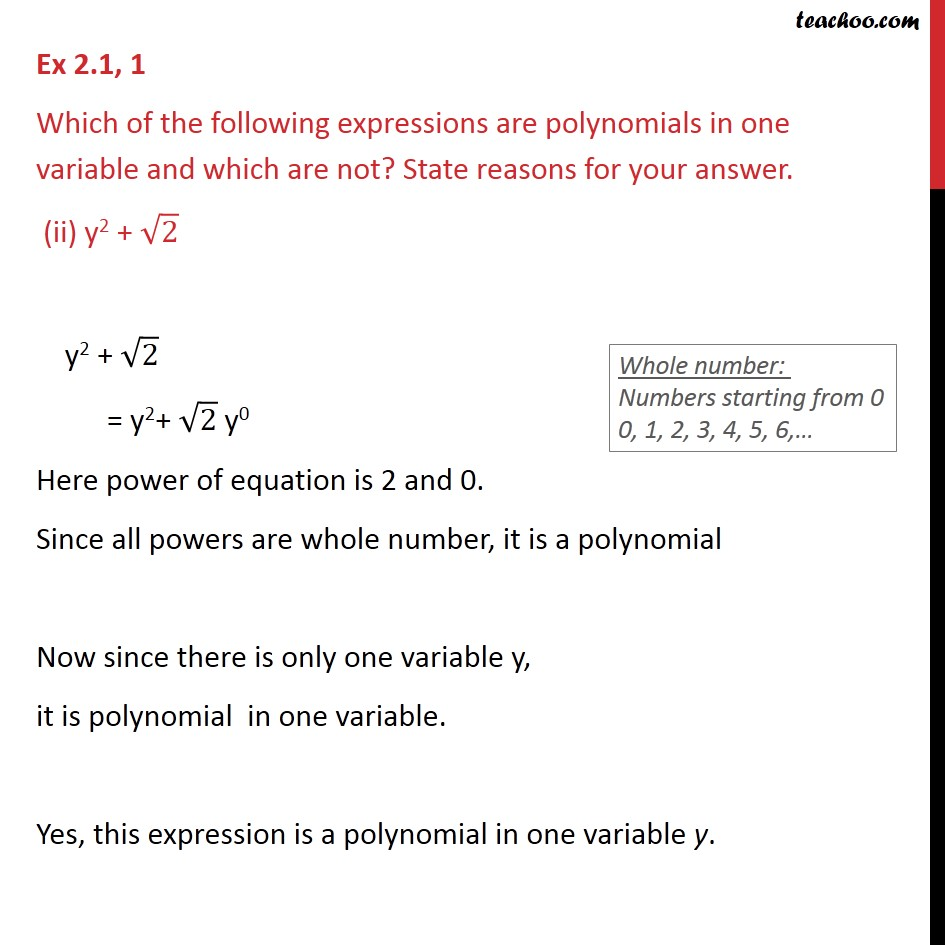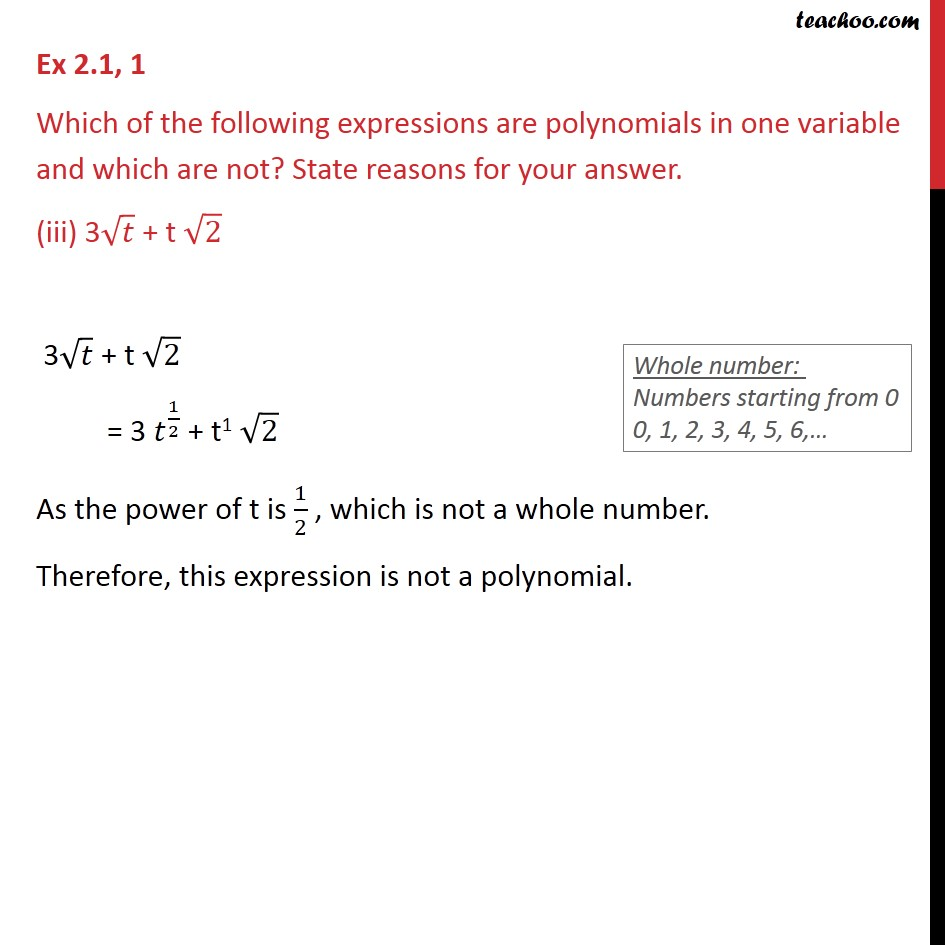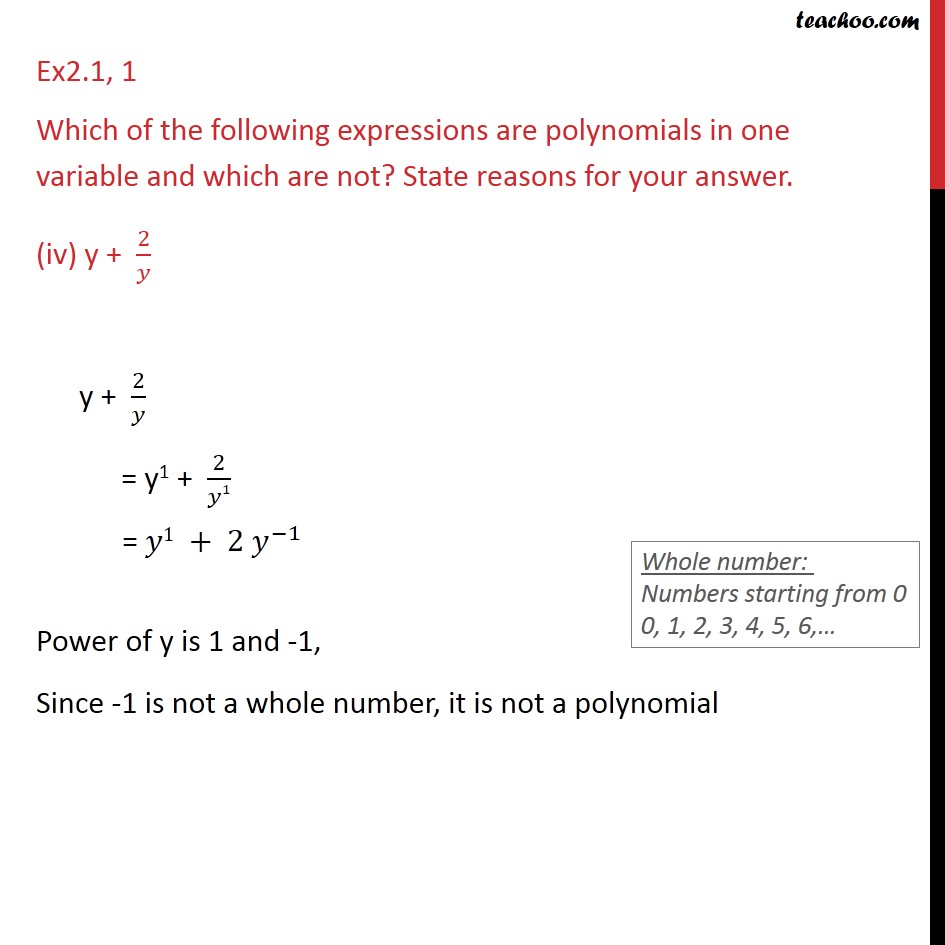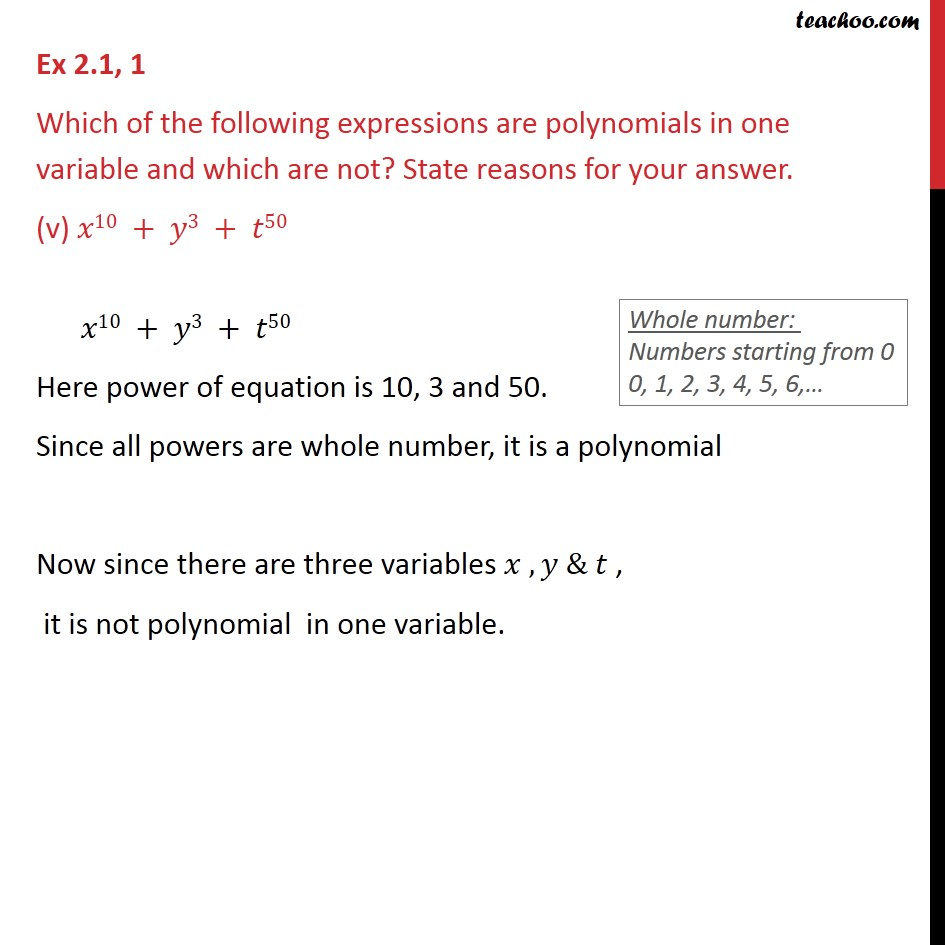1. Chapter 2 Class 9 Polynomials
2. Concept wise
3. Definition

Transcript

Ex 2.1, 1 Which of the following expressions are polynomials in one variable and which are not? State reasons for your answer. (i) 4𝑥2 – 3𝑥 + 7 4𝑥2 – 3𝑥 + 7 = 4𝑥2 – 3𝑥1 + 7 𝑥0 Here power of equation is 2,1 and 0. Since all powers are whole number, it is a polynomial Now since there is only one variable x, it is polynomial in one variable Yes, this expression is a polynomial in one variable x. Ex 2.1, 1 Which of the following expressions are polynomials in one variable and which are not? State reasons for your answer. (ii) y2 + √2 y2 + √2 = y2+ √2 y0 Here power of equation is 2 and 0. Since all powers are whole number, it is a polynomial Now since there is only one variable y, it is polynomial in one variable. Yes, this expression is a polynomial in one variable y. Ex 2.1, 1 Which of the following expressions are polynomials in one variable and which are not? State reasons for your answer. (iii) 3√𝑡 + t √2 3√𝑡 + t √2 = 3 𝑡^(1/2) + t1 √2 As the power of t is 1/2 , which is not a whole number. Therefore, this expression is not a polynomial. Ex2.1, 1 Which of the following expressions are polynomials in one variable and which are not? State reasons for your answer. (iv) y + 2/𝑦 y + 2/𝑦 = y1 + 2/𝑦1 = 𝑦1 + 2 𝑦^(−1) Power of y is 1 and -1, Since -1 is not a whole number, it is not a polynomial Ex 2.1, 1 Which of the following expressions are polynomials in one variable and which are not? State reasons for your answer. (v) 𝑥10 + 𝑦3 + 𝑡50 𝑥10 + 𝑦3 + 𝑡50 Here power of equation is 10, 3 and 50. Since all powers are whole number, it is a polynomial Now since there are three variables 𝑥 , 𝑦 & 𝑡 , it is not polynomial in one variable.

Definition